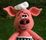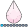## Native SAS/STAT support for the MFP (Multivariable Fractional Polynomial) selection method

When it comes to fitting multivariable regression models (linear, logistic, time-to-event, etc) the objective may be to find the "best" parsimonious model from a set of categorical and continuous independent variables using a stepwise variable selection method. Some of these variables may be predictive of the outcome, others may not. Similarly of the continuous covariates, some may fit adequately with a simple linear relation, others may require a polynomial transformation to best predict the outcome.

The Multivariable Fractional Polynomial (MFP) approach to model fitting is essentially a backward elimination procedure in which all effects are fit, and considered for deletion. When a continuous covariate is considered, the best-fitting fractional polynomial (FP) transformation is identified (usually with a maximum of two polynomial terms, and with powers from the set (-2, -1, -0.5, 0, 0.5, 1, 2, 3), with 0 representing a logarithm ter), and tested against a model without any term, against a model with a simple linear effect, and finally a model with a simpler FP form. This process to identify the most appropriate form (if any) can be done using a closed testing procedure outlined in the references (eg Section 3 of ).

SAS Macros are available to implement this method (described in reference ), but it would be far more preferable if regression modelling procedures (REG, LOGISTIC, PHREG, GLMSELECT) could provide this selection method natively. Those SAS procedures that support programming statements can accommodate a univariable FP selection approach where a FP has only 1 polynomial term (FP1), for example:

``````proc phreg data=analysis;
title 'Explore functional form of x, where x>=0';

fp1_n2 = 1 / (x+1)**2;
fp1_n1 = 1 / (x+1)**1;
fp1_n0_5 = 1 / sqrt(x+1);
fp1_0 =  log(x+1);
fp1_0_5 =  sqrt(x);
fp1_1 =  x;
fp1_2 =  x**2;
fp1_3 =  x**3;

model	Time*event(0) =fp1_n2 fp1_n1 fp1_n0_5 fp1_0 fp1_0_5 fp1_1 fp1_2 fp1_3	/ details selection=FORWARD stop=1;
run;
quit;``````

... but fitting a "univariable" FP model where the polynomial has two polynomial terms requires fitting all models with 2 of the above terms. What's more, the set of two-term FP models (FP2) includes models with repeated powers, specified in the form beta1 * x^p1 + beta2 * (x^p1) * log(x), so adjusting the above step to force exactly two effects into the final model excludes this set of FP2 models with repeated powers. The full set of FP2 transformations might be specified one by one in the programming statements as done above...

``````proc phreg data=analysis;
title 'Explore functional form of x, where x>=0';

fp2_n2_n2 = 1 / (x+1)**2 + log(x) * 1 / (x+1)**2;
fp2_n1_n2 = 1 / (x+1)**1 +```` 1 / (x+1)**2;``...````

however the procedure would fit the model beta1 * (x^p1 + x^p2), rather than the required model beta1 * x^p1 + beta2 * (x^p2) (in the case where p1 =/= p2).

References:

Regression using fractional polynomials of continuous covariates - parsimonious parametric modellingApplied Statistics 200643(3😞 429467.

 Multivariable model-building : a pragmatic approach to regression analysis based on fractional polynomials for modelling continuous variables. Patrick Royston, Willi Sauerbrei. Wiley, 2008. ISBN 9780470028421

 Morris, T. P., White, I. R., Carpenter, J. R., Stanworth, S. J., and Royston, P. (2015Combining fractional polynomial model building with multiple imputationStatist. Med.34: 3298–3317. http://dx.doi.org/10.1002/sim.6553

 W. Sauerbrei, C. Meier-Hirmer, A. Benner, P. Royston, Multivariable regression model building by using fractional polynomials: Description of SAS, STATA and R programs, Computational Statistics & Data Analysis, Volume 50, Issue 12, 2006, Pages 3464-3485,
http://dx.doi.org/10.1016/j.csda.2005.07.015

1 CommentBarite | Level 11

Three years later, is it possible to fit fractional polynomials to model terms from inside these procedures? I would like to test FP2 transformations on a term for logistic regression. If not, what would be the next best option? Were there any developments since Bruce Lund’s  macro from 2018?:

https://www.sas.com/content/dam/SAS/support/en/sas-global-forum-proceedings/2018/2390-2018.pdf

Thank you!